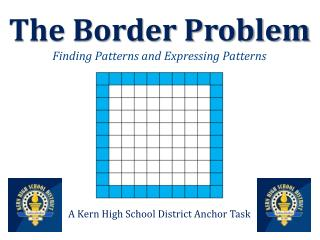DownloadDownload PresentationThe Border Problem Finding Patterns and Expressing Patterns

# The Border Problem Finding Patterns and Expressing Patterns

Télécharger la présentation## The Border Problem Finding Patterns and Expressing Patterns

- - - - - - - - - - - - - - - - - - - - - - - - - - - E N D - - - - - - - - - - - - - - - - - - - - - - - - - - -
##### Presentation Transcript

1. The Border ProblemFinding Patterns and Expressing Patterns A Kern High School District Anchor Task

2. Act 1

3. Given: the 10 by 10 grid below 1. Figure out (without talking, without writing, and without counting one-by-one) how many tiles are in the BORDER (the shaded portion) of the 10 by 10 grid above.

4. Classroom Discussion How many tiles are in the BORDER? Explain how you came up with your answer.

5. The 10 by 10 grid below represents the8th figure in a sequence of similar figures. 2. Guess how many tiles are in the BORDERof the 20th figure.Explain how you determined your guess.

6. Act 2

7. Below are the first three figures of this sequence.

8. Below are the first three figures of this sequence.

9. Below are the first three figures of this sequence.

10. Below are the first three figures of this sequence. 1. Sketch the fourth, fifth, and sixth figure.

11. Below are the first three figures of this sequence. 2. Given what you now know about the sequence, do you want to revise your guess of how many tiles are in the border of the 20th figure? If so, explain how you determined your new guess. If not, explain why.

12. 3. Explain how someone could determine the total number of tiles in the border of the 132nd figure if the 131st figure had 528 tiles in the border. 8th Figure

13. 4. Explain how someone could determine the total number of tiles in the border of the 132nd figure if they did not know the number of tiles in the border of the 131st figure. 8th Figure

14. 5. Which figure number in the sequence would contain 104 border tiles? Explain how you determined your answer. 8th Figure

15. Act 3

16. any such figure ... ... 1 2 3 8 ?

17. Explain how someone could determine the total number of border tiles in any such figure if the person knew the figure number. ?